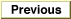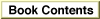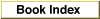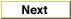# Legacy DocumentImportant: The information in this document is obsolete and should not be used for new development.

Inside Macintosh: QuickDraw GX Environment and Utilities /

# Chapter 8 - QuickDraw GX Mathematics

This chapter describes QuickDraw GX number formats, number-format conversions, mathematical functions, and functions that operate on mappings (transformation matrices). Read this chapter if your application requires the explicit use of any of the mathematical capabilities of QuickDraw GX.

Related information on how QuickDraw GX uses mappings can be found in the chapter "Transform Objects" and the chapter "View-Related Objects" in Inside Macintosh: QuickDraw GX Objects.

This chapter first describes the number formats used in QuickDraw GX. It then describes the number-format conversion macros and mathematical functions that are provided by QuickDraw GX. It then shows how to use QuickDraw GX macros and functions to provide

• fixed-point number conversions
• fixed-point operations
• operations on 64-bit numbers
• vector operations
• Cartesian and polar coordinate conversions
• random number generation
• roots of linear and quadratic equations
• bit analysis
• mapping operations

Chapter Contents
Number Formats
Integer Formats
Floating-Point Formats
Fixed-Point Formats
Working With Bias in Fixed-Point Operations
Number-Conversion Macros
Mathematical Functions
Operations on Fixed, long, and fract Numbers
Operations on wide Numbers
Vector Operations
Cartesian and Polar Coordinate Conversion
Random Number Generation
Roots of Linear and Quadratic Equations
Bit Analysis
Transformation Operations With Mappings
Characteristics of a Mapping
Translation by a Relative Amount
Translation to a Specified Point
Scaling
Rotation
Skewing
Perspective
Using QuickDraw GX Mathematics
Converting Number Formats
Performing Fixed-Point Operations
Converting Between Cartesian and Polar Coordinates
Performing Vector Operations
Shifting the Bits of a wide Number
Determining the Highest Order Bit of a wide Number
Generating Random Numbers
Analyzing the Bits in a Number
Resetting a Mapping
QuickDraw GX Mathematics Reference
Constants and Data Types
Number Formats and Constants
The Mapping Structure
Number-Conversion Macros
Format Conversions
Rounding, Truncating, and Square Root Operations
Mathematical Functions
Fixed-Point Operations
Operations on wide Numbers
Vector Operations
Cartesian and Polar Coordinate Point Conversions
Random Number Generation
Bit Analysis
Mapping Functions
Manipulating and Applying Mappings
Modifying Mappings
Summary of QuickDraw GX Mathematics
Constants and Data Types
Number Formats and Constants
The Mapping Structure
Number-Conversion Macros
Format Conversions
Rounding, Truncating, and Square Root Operations
Mathematical Functions
Fixed-Point Operations
Operations on wide Numbers
Vector Operations
Cartesian and Polar Coordinate Point Conversions
Random Number Generation